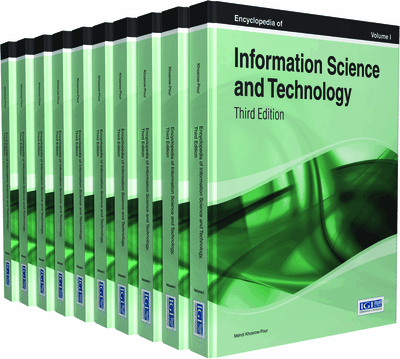# Forecasting Techniques for Energy Optimization in Buildings

Fernando Mateo (University of Valencia, Spain), Juan José Carrasco (University of Valencia, Spain), Abderrahim Sellami (University of Valencia, Spain), Mónica Millán-Giraldo (University of Valencia, Spain), Manuel Domínguez (University of León, Spain), Ignacio Díaz (University of Oviedo, Spain) and Emilio Soria-Olivas (University of Valencia, Spain)
DOI: 10.4018/978-1-4666-5888-2.ch092
Available
\$37.50
No Current Special Offers

Top

## Background

Forecasting applications often rely on classical linear statistical modeling techniques like Multiple Linear Regression (MLR), Robust Multiple Linear Regression (RMLR) (Weisberg, 1985) and ARMA models (Makridakis et al., 1998). More recently, machine learning methods such as Artificial Neural Networks (ANNs) (Eynard et al., 2011) or Support Vector Machines (SVMs) (Li et al., 2009), have emerged as a robust alternative to classical models, generally enabling a better approximation of complex, non-linear relationships between the system inputs and outputs. ANNs cover a wide range of forecasting problems, and they are known for their flexibility and high predictive accuracy (Haykin, 2008). Therefore, they have a widespread use in the field of building indoor temperature prediction (Gouda et al., 2002; Mustafaraj et al., 2011; Ruano et al., 2006).

In the literature it is possible to find comparisons of linear models with ANNs applied to daily temperature profile forecast (Hippert & Pedreira, 2004) and indoor temperature forecast in buildings (Mechaqrane & Zouak, 2004; Thomas & Soleimani-Mohseni, 2007; Mateo et al., 2012). These studies conclude that ANNs are more advantageous than linear models. In the field of power load forecasting, one can find time series approaches (Paarmann & Najar, 1995), regression analysis (Goia et al., 2010), ANNs (Wang et al., 2012) and, more recently, SVMs (Zheng et al., 2011).

In this chapter, we introduce a variety of advanced forecasting techniques like Extreme Learning Machines (ELMs) (Huang et al., 2006), Multilayer Perceptrons (MLPs), Least-Squares-Support Vector Machines (LS-SVM) (Suykens et al., 2002), General Regression Neural Networks (GRNNs) (Specht, 1991) and a combination of MLPs with Non-linear Autoregressive Exogenous techniques (NARX) (Lentaritidis & Billings, 1985a; Lentaritidis & Billings, 1985b). These methods are going to be evaluated on two applications related to energy optimization in buildings, more precisely:

• Forecast of the next-hour indoor temperature fluctuation from data obtained from a building simulator. For this we use the record of past temperatures and other environmental variables.

• Short-term (next hour) electrical load consumption forecasting from data acquired from a set of buildings in the University of León (Spain).

## Key Terms in this Chapter

Forecasting: Predicting the future values of the response variables of a system. For example, making predictions about the weather or the stock market evolution.

Time Series: An ordered sequence of values that correspond to a variable that is typically sampled at a uniform sampling rate. Time series prediction is intended to make estimations about the future values of the series.

Machine Learning: A branch of artificial intelligence related to the design of algorithms that learn dynamically from examples of their input variables to make estimations about the output ones.

Autoregressive Model: Random process which is often used to predict the future values of a time series as a function of its previous values. Autoregressive methods can be linear or non-linear, and can be univariate or use more than one input variable to help the prediction.

Artificial Neural Network: Adaptive mathematical model based on the interconnection of elements, called artificial neurons, that aims to obtain an estimation of the output variables of the system by learning from given examples of the input variables. They can be used for function approximation, classification, pattern recognition, etc.

Multiple Linear Regression: Modelling technique that consists of fitting a linear equation that provides the best adjustment between two or more input variables and a response variable.

Energy Efficiency: Minimization of the energy consumption while keeping the same energetic service. In buildings, this applies to the energy management (heating, air conditioning, ventilation) that provides the optimal energy consumption while keeping reasonable comfort conditions for the occupants.

## Complete Chapter List

Search this Book:
Reset